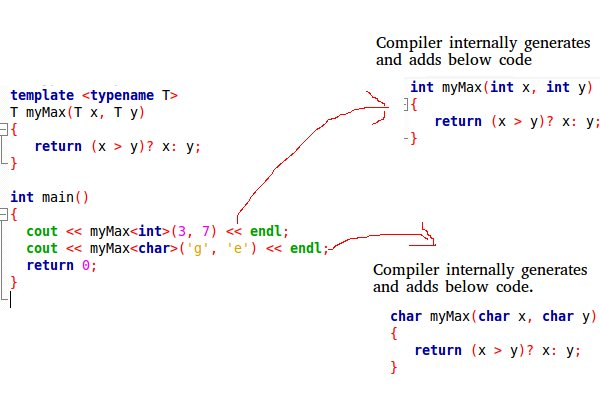Templates in C++

• Difficulty Level : Medium
• Last Updated : 28 Jun, 2021

A template is a simple and yet very powerful tool in C++. The simple idea is to pass data type as a parameter so that we don’t need to write the same code for different data types. For example, a software company may need sort() for different data types. Rather than writing and maintaining the multiple codes, we can write one sort() and pass data type as a parameter.
C++ adds two new keywords to support templates: ‘template’ and ‘typename’. The second keyword can always be replaced by keyword ‘class’.
How do templates work?
Templates are expanded at compiler time. This is like macros. The difference is, the compiler does type checking before template expansion. The idea is simple, source code contains only function/class, but compiled code may contain multiple copies of same function/class.Function Templates We write a generic function that can be used for different data types. Examples of function templates are sort(), max(), min(), printArray().
Know more on Generics in C++

CPP

 #include using namespace std; // One function works for all data types.  This would work// even for user defined types if operator '>' is overloadedtemplate T myMax(T x, T y){   return (x > y)? x: y;} int main(){  cout << myMax(3, 7) << endl;  // Call myMax for int  cout << myMax(3.0, 7.0) << endl; // call myMax for double  cout << myMax('g', 'e') << endl;   // call myMax for char   return 0;}

Output:

7
7
g

Below is the program to implement Bubble Sort using templates in C++:

CPP

 // CPP code for bubble sort// using template function#include using namespace std;  // A template function to implement bubble sort.// We can use this for any data type that supports// comparison operator < and swap works for it.template void bubbleSort(T a[], int n) {    for (int i = 0; i < n - 1; i++)        for (int j = n - 1; i < j; j--)            if (a[j] < a[j - 1])              swap(a[j], a[j - 1]);}  // Driver Codeint main() {    int a = {10, 50, 30, 40, 20};    int n = sizeof(a) / sizeof(a);      // calls template function    bubbleSort(a, n);      cout << " Sorted array : ";    for (int i = 0; i < n; i++)        cout << a[i] << " ";    cout << endl;    return 0;}

Output

Sorted array : 10 20 30 40 50

Class Templates Like function templates, class templates are useful when a class defines something that is independent of the data type. Can be useful for classes like LinkedList, BinaryTree, Stack, Queue, Array, etc.
Following is a simple example of template Array class.

CPP

 #include using namespace std; template class Array {private:    T *ptr;    int size;public:    Array(T arr[], int s);    void print();}; template Array::Array(T arr[], int s) {    ptr = new T[s];    size = s;    for(int i = 0; i < size; i++)        ptr[i] = arr[i];} template void Array::print() {    for (int i = 0; i < size; i++)        cout<<" "<<*(ptr + i);    cout< a(arr, 5);    a.print();    return 0;}

Output:

1 2 3 4 5

Can there be more than one arguments to templates?
Yes, like normal parameters, we can pass more than one data types as arguments to templates. The following example demonstrates the same.

CPP

 #includeusing namespace std; templateclass A  {    T x;    U y;public:    A() {    cout<<"Constructor Called"< a;   A b;   return 0;}

Output:

Constructor Called
Constructor Called

Can we specify default value for template arguments?
Yes, like normal parameters, we can specify default arguments to templates. The following example demonstrates the same.

CPP

 #includeusing namespace std; templateclass A  {public:    T x;    U y;    A() {   cout<<"Constructor Called"< a;  // This will call A     return 0;}

Output:

Constructor Called

Both function overloading and templates are examples of polymorphism feature of OOP. Function overloading is used when multiple functions do similar operations, templates are used when multiple functions do identical operations.
What happens when there is a static member in a template class/function?
Each instance of a template contains its own static variable. See Templates and Static variables for more details.
What is template specialization?
Template specialization allows us to have different code for a particular data type. See Template Specialization for more details.
Can we pass nontype parameters to templates?
We can pass non-type arguments to templates. Non-type parameters are mainly used for specifying max or min values or any other constant value for a particular instance of a template. The important thing to note about non-type parameters is, they must be const. The compiler must know the value of non-type parameters at compile time. Because the compiler needs to create functions/classes for a specified non-type value at compile time. In below program, if we replace 10000 or 25 with a variable, we get a compiler error. Please see this.
Below is a C++ program.

CPP

 // A C++ program to demonstrate working of non-type// parameters to templates in C++.#include using namespace std;  template int arrMin(T arr[], int n){   int m = max;   for (int i = 0; i < n; i++)      if (arr[i] < m)         m = arr[i];     return m;}  int main(){   int arr1[]  = {10, 20, 15, 12};   int n1 = sizeof(arr1)/sizeof(arr1);     char arr2[] = {1, 2, 3};   int n2 = sizeof(arr2)/sizeof(arr2);     // Second template parameter to arrMin must be a constant   cout << arrMin(arr1, n1) << endl;   cout << arrMin(arr2, n2);   return 0;}

Output:

10
1

What is template metaprogramming?
See Template Metaprogramming
You may also like to take a quiz on templates.
Java also supports these features. Java calls it generics .